### Choose language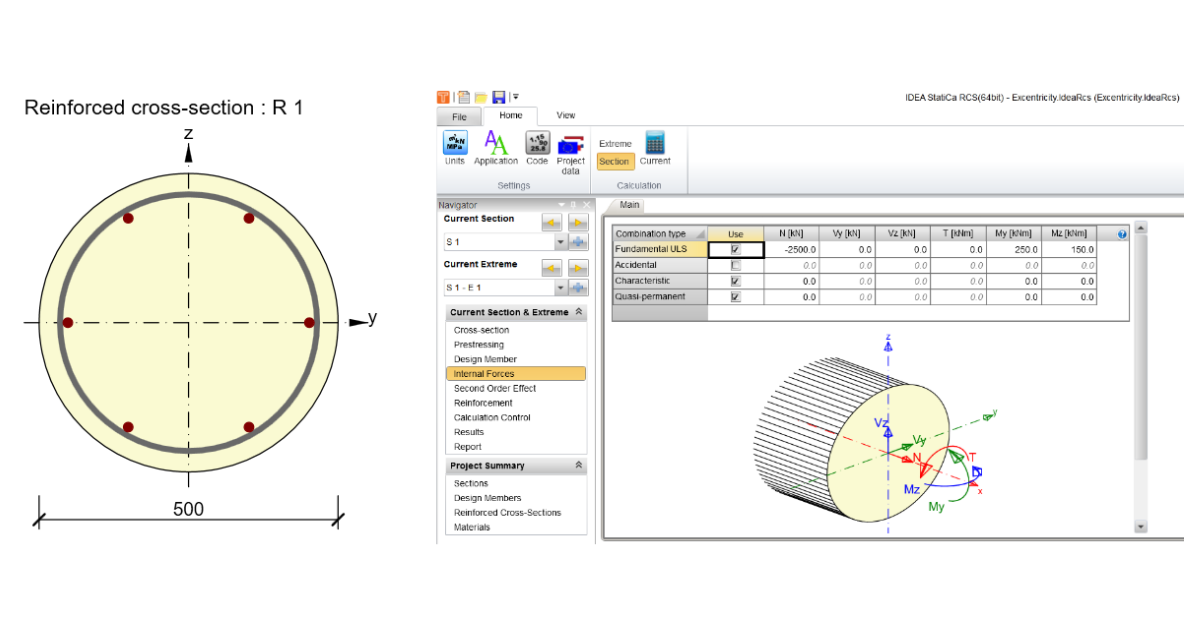# How to calculate resultant first order eccentricity on the compression member

$$A compression member is subjected by normal force and bending moments in both planes y,z. The resultant first order eccentricity is calculated as the ratio of a design normal force and bending moments plus geometrical eccentricity counted as the length of a column/ 400 for a single column.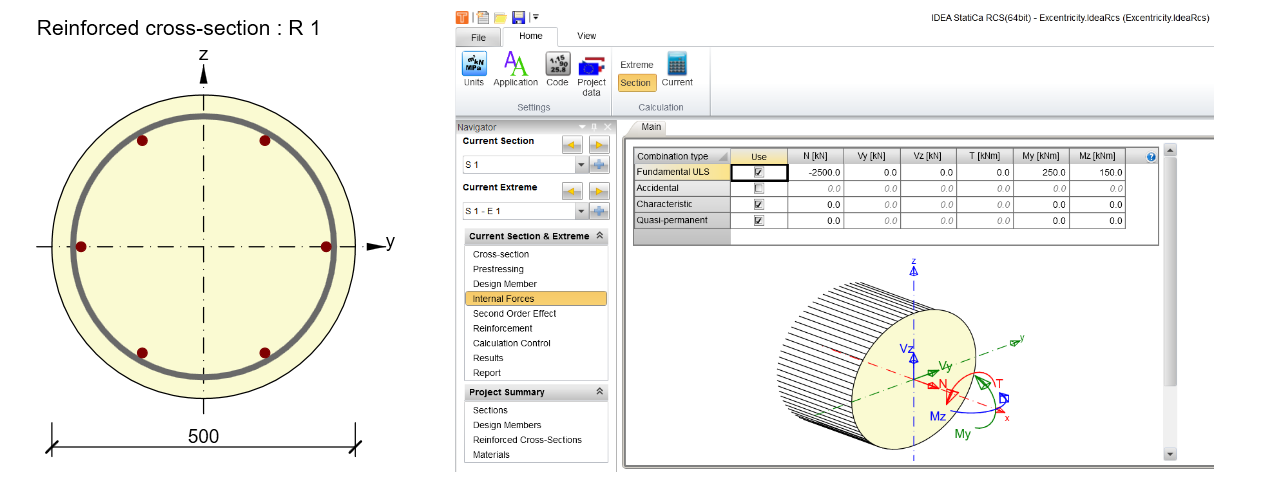Calculation of a first-order eccentricity and imperfectionsIn the navigator tree, there is the Second Order Effect tab which serves for calculation resultant first order and second-order effect on the column.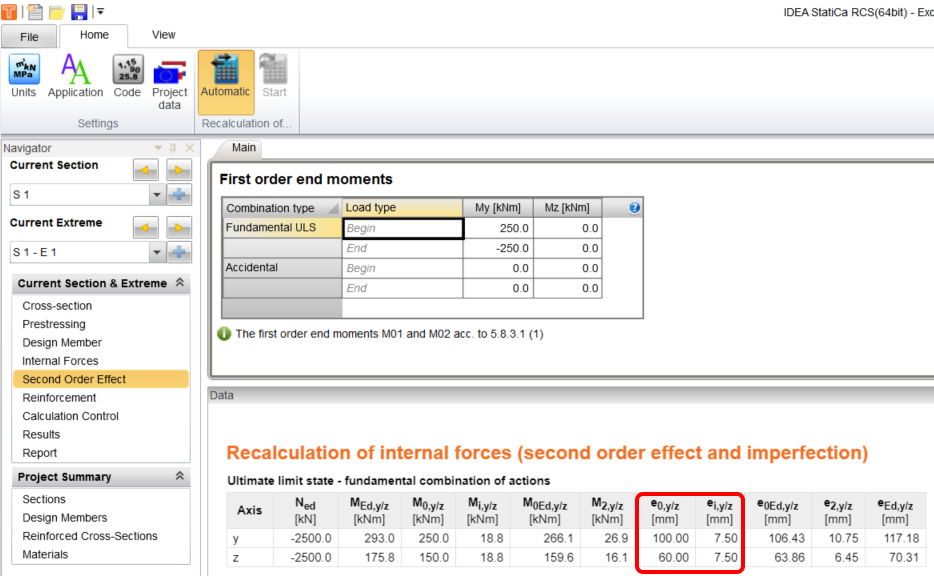First order eccentricity :e0,y/z=M0,y/z/Ned=250/2500 = 100 mme0,y/z=M0,y/z/Ned=150/2500 = 60 mmImperfections :eiy/z=l0/400 = 3000/400 =7.5 mmCalculation of a resultant first order eccentricityThe resultant eccentricity e0ed,yzis counted as first order eccentricitye0,y/zplus increment geometrical imperfection eiy/zto each direction.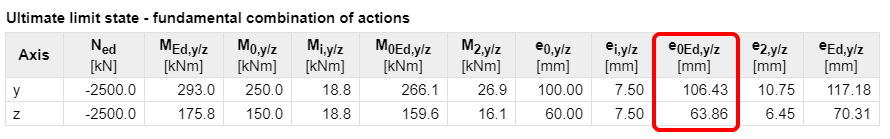arctg(α) = e0,y/e0,z= 100/60 = 59.03 °ei,y = sin (α) * ei,y/z=sin (59.03)*7.5 = 6.43 mmei,z= cos (α) * ei,y/z=cos (59.03)*7.5 = 3.86mmResultant first order eccentricity:e0Ed,y = e0,y + ei,y = 100 + 6.43 = 106.43 mme0Ed,z = e0,z + ei,z = 60+ 3.86 = 106.43 mm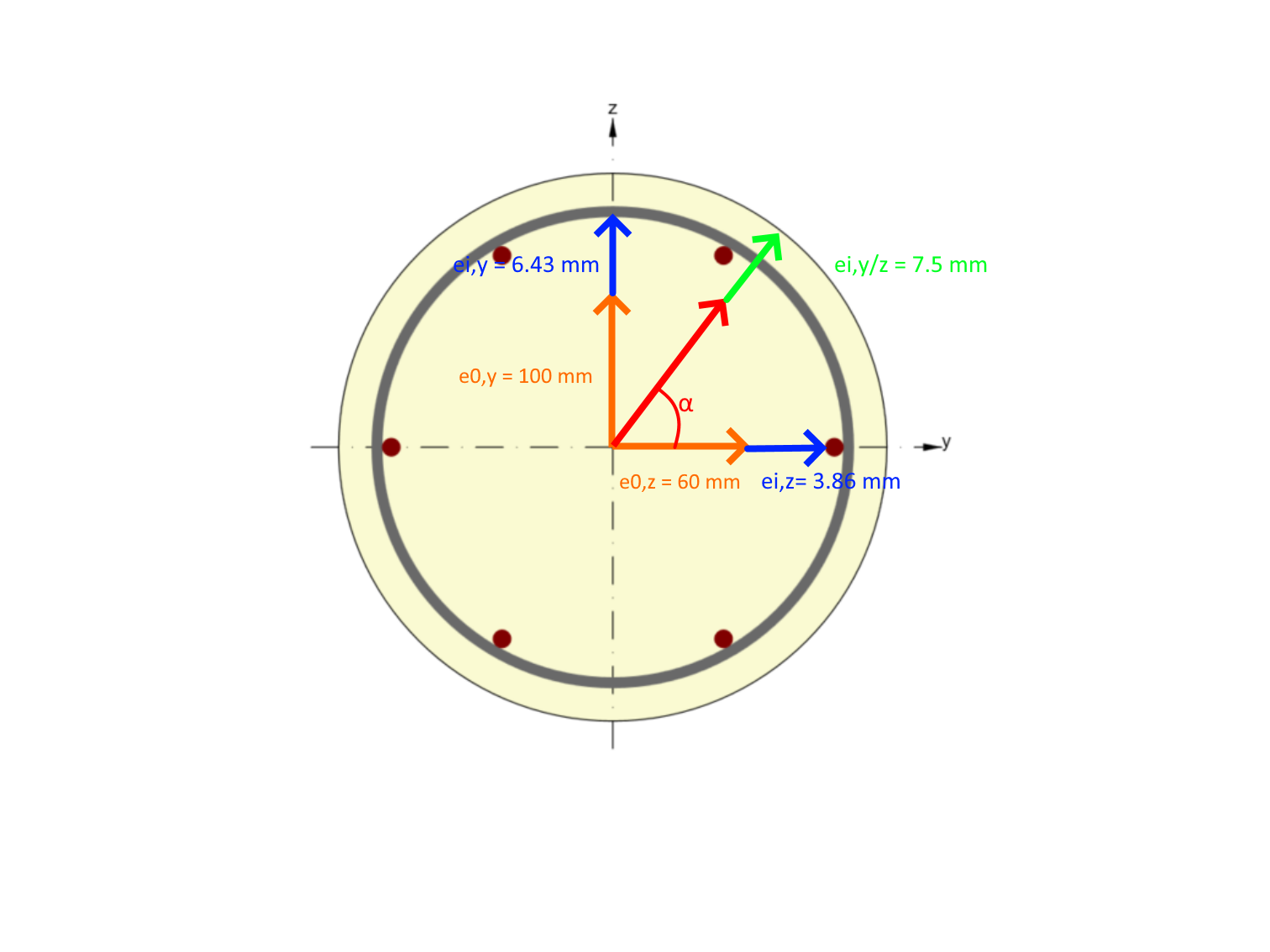$$